# IIT JEE Mathematics Study Notes

“Without mathematics, there’s nothing you can do , everything around you is mathematics.
Everything around you is numbers.” - Shakuntala Devi

Mathematics is the language of science. And every aspiring engineer is expected to achieve fluency in it. In IIT JEE , 30 questions out of a total of 90 , worth 120 marks come from the maths section. It is scoring if one is proficient in it , or else it can act as the tie-breaker.

CLEAR EXAM hopes to be a guiding light in this respect, by providing the students with detailed IIT JEE Mathematics study notes, practice exercises and solved examples on all the important topics of maths for the IIT JEE exam.

To be helpful further, we would like to share with you a descriptive analysis of which topics are crucial wrt IIT JEE.

Crucial topics : Definite Integral and the area under the curve, limits, continuity and differentiability, functions, applications of derivatives, conic sections, complex numbers, quadratic equation, binomial theorem and MI, trigonometric ratios and functions, properties of a triangle, permutation and combination, indefinite integral.

We hope that our notes will play their part in our students' success.

Success in the IIT JEE exam requires a strong foundation in mathematics, as it is an integral part of the syllabus. To support your exam preparation, we have developed a comprehensive collection of IIT JEE mathematics study notes and short guides. Our study material is designed to help you grasp essential concepts, master problem-solving techniques, and build confidence for the exam. Let's explore the key features of our JEE maths study notes.

Comprehensive Coverage of Key Topics: Our study notes cover the entire mathematics syllabus of the IIT JEE exam, leaving no stone unturned. From algebra, calculus, and coordinate geometry to trigonometry, statistics, and probability, our notes provide a thorough understanding of each topic. We ensure that you have access to clear explanations, relevant examples, and concise summaries for every concept, allowing you to consolidate your knowledge effectively.

Condensed and Organized Maths Short Notes: We understand the need for quick revision during the exam preparation phase. Hence, we have carefully condensed the study material into short notes, providing you with a handy reference guide. Our IIT JEE Mathematics short notes are organized chapter-wise, enabling you to navigate through different topics effortlessly. With our concise and structured approach, you can easily locate specific information when revisiting key concepts.

Effective Problem-Solving Techniques: Mathematics requires proficiency in problem-solving, and our IIT JEE maths study notes focus on equipping you with effective techniques. We provide step-by-step solutions to a wide range of practice questions, allowing you to understand the problem-solving process thoroughly. By practicing these questions, you will enhance your analytical skills, speed, and accuracy in solving mathematical problems—a crucial advantage during the exam.

Visual Representation and Diagrams: Visual aids play a significant role in understanding complex mathematical concepts. Our IIT JEE Mathematics study notes incorporate diagrams, graphs, and visual representations wherever applicable to facilitate better comprehension. We believe that visualizing mathematical relationships and representations improves your understanding and retention of the subject matter.

Regular Updates: Keeping up with the evolving IIT JEE syllabus is essential, and our study notes are regularly updated to reflect any changes. We ensure that our content aligns with the latest examination pattern and requirements. By relying on our up-to-date study material, you can stay ahead of the competition and focus on mastering the relevant topics.

IIT JEE Mathematics Study Notes | JEE Maths Short Notes PDF

Conclusion: A strong foundation in mathematics is key to excelling in the IIT JEE exam. Our meticulously crafted study notes and short guides offer comprehensive coverage, effective problem-solving techniques, and concise summaries of essential mathematical concepts. By leveraging our study material, you can streamline your preparation, strengthen your understanding, and maximize your chances of success in the IIT JEE mathematics section. Start your journey towards mathematical excellence today with our top-notch IIT JEE mathematics study notes.

Mathematics
Sets, Relations and FunctionsMathematics
Trigonometric Ratios and Identities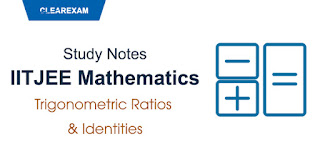Mathematics
Trigonometric Equations & Inequations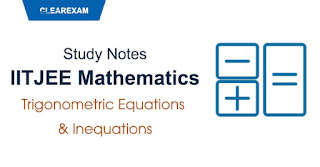Mathematics
Properties of Triangles, Height & DistancesMathematics
Principles of Mathematical Induction, Permutation & CombinationMathematics
Complex Numbers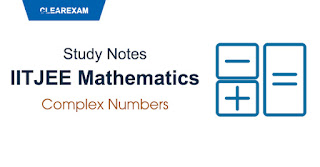MathematicsMathematics
Linear InequalitiesMathematics
Sequence and SeriesMathematics
Binomial Theorem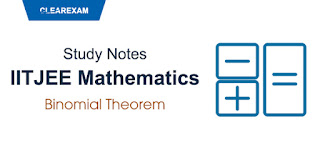Mathematics
Infinite SeriesMathematics
Points & Straight Lines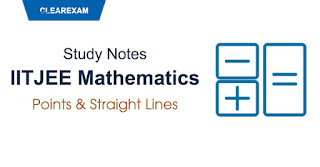Mathematics
Pair of Straight LinesMathematics
CirclesMathematics
ParabolaMathematics
Ellipse & Hyperbola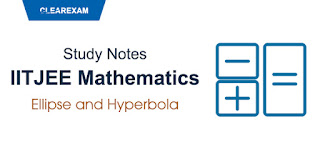Mathematics
Mathematical Reasoning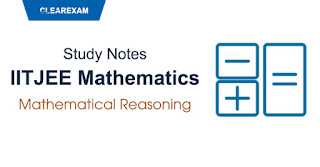Mathematics
Statistics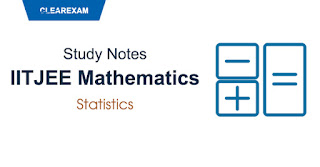Mathematics
Probability BasicMathematics
FunctionsMathematics
Inverse Trigonometric Functions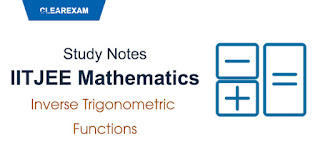Mathematics
Matrices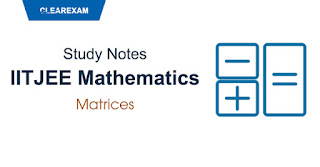Mathematics
DeterminantsMathematics
Limits, Continuity and Differentiability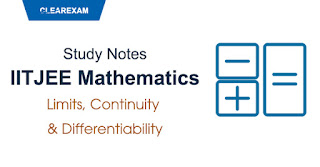Mathematics
Differentiation & Application of DerivativesMathematics
Indefinite Integration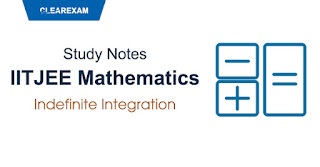Mathematics
Definite Integration & ApplicationsMathematics
Differential Equations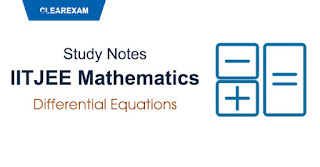Mathematics
Vector Algebra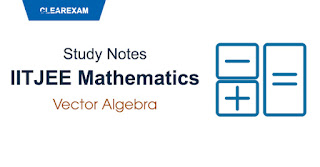Mathematics
Three Dimensional Geometry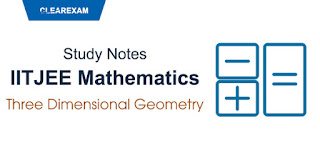Mathematics## Want to know more

Please fill in the details below:

## Latest NEET Articles\$type=three\$c=3\$author=hide\$comment=hide\$rm=hide\$date=hide\$snippet=hide

Name

ltr
static_page
BEST NEET COACHING CENTER | BEST IIT JEE COACHING INSTITUTE | BEST NEET & IIT JEE COACHING: IIT JEE Mathematics Study Notes PDF – Summary, Important Formula | Maths Short Notes for IIT JEE Preparation, Free Download
IIT JEE Mathematics Study Notes PDF – Summary, Important Formula | Maths Short Notes for IIT JEE Preparation, Free Download
Gain a competitive edge in your IIT JEE exam with meticulously crafted Mathematics study notes. Download free JEE Maths short notes.
BEST NEET COACHING CENTER | BEST IIT JEE COACHING INSTITUTE | BEST NEET & IIT JEE COACHING
https://www.cleariitmedical.com/p/iit-jee-mathematics-study-notes.html
https://www.cleariitmedical.com/
https://www.cleariitmedical.com/
https://www.cleariitmedical.com/p/iit-jee-mathematics-study-notes.html
true
7783647550433378923
UTF-8

STAY CONNECTED